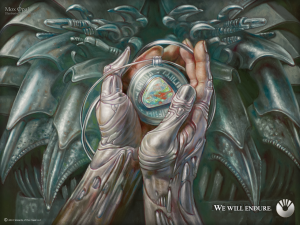# Wacky Python Image Creation

The other night I had a wacky idea of extracting each pixel from an image in order to save it as a plain text ASCII file.

Of course this is not ideal and can take a bit of time, but like most things I do with python its just for the fun of it.

I figured the easiest way to achieve this would be to use Python’s Image library and save the output to a serialized pickle text file.

I began to write a simple piece of code to extract each pixel from an image.

First off we need to import a few external libraries to assist:

#!/usr/bin/env python
import Image
import argparse
import pickle


In order to give us some useful –help output I used argparse to take input:

parser = argparse.ArgumentParser()
args = parser.parse_args()


Once we have our file name we can load the image using PIL and grab the size of image:

im = Image.open(args.filename)
width, height = im.size


Here we have to have some way to keep track of our Y and X Axis of pixels, we can do this with a couple strings:

y_cursor = 0
x_cursor = 0


We will need something to hold the data we collect, why not a list:

image = []


This block is a bit more complex, but all we are doing is stepping through each pixel kind of like a typewriter would do when writing a document. We are using the getpixel method from PIL to extract the pixels RGB information:

print 'Processing %s...' % args.filename
while y_cursor < height:
row = []
while x_cursor < width:
row.append(im.getpixel((x_cursor, y_cursor)))
x_cursor += 1
x_cursor = 0
y_cursor += 1
image.append(row)


And lastly we will save all the captured data to a pickle file:

f = open(args.output, 'wb')
pickle.dump(image, f)


Once this small script was created I attempted to serialize a JPEG image:The details of the image are:

$ls -lh mox.jpg -rw-r--r--@ 1 user staff 870K Nov 10 08:56 mox.jpg  And after running the above Python script I got a ASCII file of: $ ls -lh output
-rw-r--r--  1 user  staff    28M Nov 10 08:56 output


Yes, this made me chuckle. Going from 870K binary JPEG to 28M ASCII text, but as mentioned this is just a proof of concept.

The serialized data ended up being quite a few lines, 4916161 to be exact:

$wc -l output 4916161 output  Lets go ahead and take a peak at the un-serialized data: >>> import pickle >>> f = open('output', 'rb') >>> image = pickle.load(f) >>> >>> len(image) 960 >>> >>> len(image) 1280 >>> >>> image (40, 43, 34) >>> >>> image [(40, 43, 34), (39, 42, 33), (42, 45, 36), (41, 44, 35), (40, 43, 34), (38, 41, 32), .....  Now all that was left was to write another small script to take this ASCII content and turn it back in to a image. Like before we will import our libraries and use argparse to take input: #!/usr/bin/env python import Image import argparse import pickle parser = argparse.ArgumentParser() parser.add_argument('filename', help='Extracted Pickle File') parser.add_argument('output', help='Output Filename') args = parser.parse_args()  Since we have the filename from argparse we need to read it and parse it through pickle : f = open(args.filename, 'rb') image = pickle.load(f)  Using the concept of one list per row I can determine the width and height of the original image via len: height = len(image) width = len(image) size = (width, height)  Now we are ready to create the image container and reset our cursors to start position: im = Image.new('RGB', size) # define our cursors to # parse over the images pixel by pixel y_cursor = 0 x_cursor = 0  Just like before use a typewriter motion to add to each pixel, here we use the PIL method putpixel : print 'Building %s...' % args.output while y_cursor < height: while x_cursor < width: pixel = image[y_cursor][x_cursor] im.putpixel((x_cursor, y_cursor), pixel) x_cursor += 1 x_cursor = 0 y_cursor += 1  And lastly save the Image to our output file name: im.save(args.output)  And Abracadabra!$ ls -lh new_mox.png
-rw-r--r--  1 user  staff   1.7M Nov 10 09:05 new_mox.png